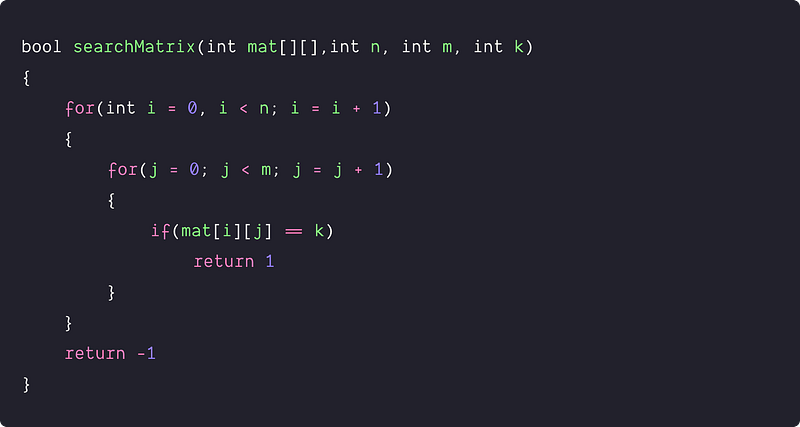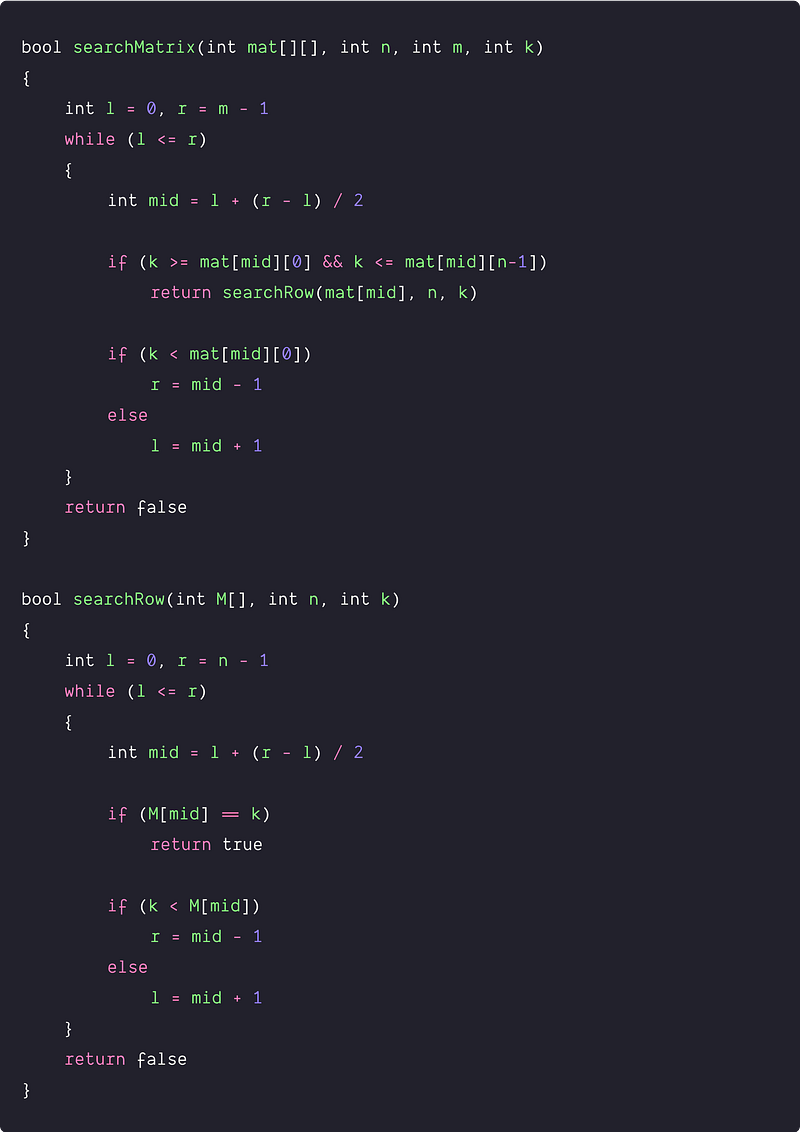# Search in a row-wise sorted 2D matrix

Difficulty: Easy, Asked-in: Microsoft, Amazon, Adobe, Directi, Goldman Sachs, Paytm, SAP, Visa

Key takeaway: An excellent problem to learn problem-solving using the binary search. We can apply binary search twice to search the value i.e. first time for reducing the search space by finding a row and the second time for searching in that row. Think!

### Let's understand the problem

You are given a row-wise sorted 2D matrix and a given integer k, write a program to find whether the integer ‘k’ is present in the matrix or not. The matrix has the following properties:

• Integers in each row are sorted from left to right.
• The first integer of each row is greater than the last integer of the previous row.

Examples

``````Input: k = 6
mat[][] =
[
[1,   2,  6,  7],
[12, 13, 16, 21],
[23, 35, 36, 48]
]

Output: true
Explanation: value k = 6 is present in the matrix.

Input: k = 7
mat[][] =
[
[1,   2,  6],
[12, 13, 16],
[23, 35, 36]
]

Output: fasle
Explanation: value k = 7 is not present in the matrix.
``````

### Discussed solution approaches

• A brute force approach  using nested loops
• An efficient approach  using binary Search

### A brute force approach using nested loops

A simple approach would be to traverse the whole matrix using nested loops and check whether the k is present or not.

Solution PseudocodeTime and space complexity analysis

To traverse the array, we used two loops, where the outer loop selects the row, and the inner loop keeps track of the column. Time Complexity = O(n*m), Here n is the number of rows, and m is the number of columns. Space Complexity = O(1), we are not using any additional space.

Now the critical question: how can we improve the time complexity? Can we use the sorted order property to solve it efficiently? Let's think!

### An efficient approach using binary search

Solution Idea

As we can see, each row in the matrix is sorted, and the first element of a row is greater than or equal to the last number of the preceding row. Therefore, the matrix can be viewed as a sorted one-dimensional array (both row-wise and column-wise). can we think to apply the idea of binary search? let's think!

If we pick any ith row in the middle then all the values in the ith row would be:

• Sorted in increasing order
• Greater than all the values in (i-1)th row.
• Less than all the values in (i+1)th row.

So we can use the above property and use the following idea:

• Apply binary search on matrix rows to find out the row in which ‘k’ must lie, i.e., we need to find a row such that the first element of the row is greater than or equal to ‘k’ and the last element of the row is less than or equal to ‘k.’
• Then, apply a binary search in that row to find out if ‘k’ is present in the matrix or not.

Solution PseudocodeTime and space complexity analysis

Time complexity = Time complexity of binary search to search the row + Time complexity of binary search to to search value K = O(logn) + O(logm) = O(log n+log m)
Space complexity: O(1), because we are using the iterative binary search.

### Critical ideas to think!

• Suppose our matrix is just sorted in-row and column-wise but not strictly sorted i.e — the first integer of each row is not greater than the last integer of the previous row. Can we solve the problem using the above approach of the binary search?
• How do we modify the above code, if we need to return the index (i, j) of the value k, if k is present in the matrix?
• Can we solve the above problem by searching the column and then searching elements in that column?
• How can we implement the above approach using a recursive binary search? What will be the space complexity?

### Comparisons of time and space complexities

• Using nested loops: Time = O(nm), Space = O(1)
• Using binary search: Time = O(logn + logm), Space = O(1)

### Similar problems for practice

Thanks to Navtosh Kumar for his contribution in creating the first version of the content. Please write comments if you find an error or you want to share more insights about the topic. Enjoy learning, Enjoy coding, Enjoy algorithms!

Get well-designed application and interview centirc content on ds-algorithms, machine learning, system design and oops. Content will be delivered weekly.